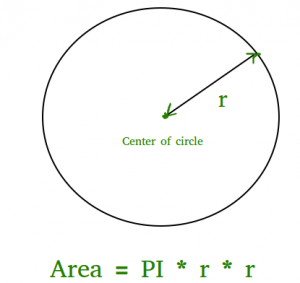# Program to find area of a circle

• Difficulty Level : Medium
• Last Updated : 06 Nov, 2021

The area of a circle can simply be evaluated using the following formula.```Area = pi * r2
where r is radius of circle and it maybe in float because value of pie is 3.14 ```

## C++

 `// C++ program to find area``// of circle``#include ``const` `double` `pi = 3.14159265358979323846;``using` `namespace` `std;` `// function to calculate the area of circle``float` `findArea(``float` `r)``{``    ``return` `(pi * r * r);``}``// driver code``int` `main()``{``    ``float` `r, Area;``    ``r = 5;` `    ``// function calling``    ``Area = findArea(r);``    ``// displaying the area``    ``cout << ``"Area of Circle is :"` `<< Area;``    ``return` `0;``}`

## C

 `// C program to find area``// of circle``#include ``#include ``#define PI 3.142` `double` `findArea(``int` `r)``{``   ``return` `PI * ``pow``(r, 2);``}` `int` `main()``{``    ``printf``(``"Area is %f"``, findArea(5));``    ``return` `0;``}`

## Java

 `// Java program to find area``// of circle``    ` `class` `Test``{``    ``static` `final` `double` `PI = Math.PI;``         ` `    ``static` `double` `findArea(``int` `r)``    ``{``       ``return` `PI * Math.pow(r, ``2``);``    ``}``         ` `    ``// Driver method``    ``public` `static` `void` `main(String[] args)``    ``{``        ``System.out.println(``"Area is "` `+  findArea(``5``));``    ``}``}`

## Python3

 `# Python3 program to find Area of a circle` `def` `findArea(r):``    ``PI ``=` `3.142``    ``return` `PI ``*` `(r``*``r);` `# Driver method``print``(``"Area is %.6f"` `%` `findArea(``5``));` `# This code is contributed by Chinmoy Lenka`

## C#

 `// C# program to find area of circle``using` `System;` `class` `GFG``{``    ``static` `double` `PI = Math.PI;``        ` `    ``static` `double` `findArea(``int` `r)``    ``{``    ``return` `PI * Math.Pow(r, 2);``    ``}``        ` `    ``// Driver method``    ``static` `void` `Main()``    ``{``        ``Console.Write(``"Area is "` `+ findArea(5));``    ``}``}` `// This code is contributed by Sam007.`

## PHP

 ``

## Javascript

 ``

Output:

`Area is 78.550000`

Time Complexity: O(1)

Auxiliary Space: O(1)

My Personal Notes arrow_drop_up